IF YOU CAN SEE THIS go to /sfa/Nuprl/Shared/Xindentation_hack_doc.html

Thm*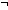(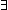A:Type, Q:(A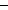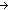AProp).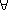P:(AProp).p:Ax:AQ(x,p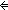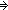P(x))

Often Russell's Paradox is phrased in terms of sets and the membership relation between sets.

Let "x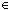y" represent membership of one set in another. Russell's argument is paradoxical when we accept as principle the claim thatP:(SetProp).p:Setx:Set. (xpP(x)

which is sometimes called "unlimited comprehension", and may seem quite plausible.

Notice that unlimited comprehension is an instance of the body of the above theorem, using Set for A, and "xp" for Q(x,p), and so is false. But when one mistakes it for being true, it leads top:Setx:Set. (xp(xx)

by letting P(x) be(xx), which in turn allows us to make the disturbing conclusion:p:Set. (pp(pp),

(see lemma Thm*(PP)).

This contradiction does not depend essentially on the fact that we are talking about sets and trying to represent each property of sets as the set of all sets with that property.

Abstracting away from these details, we get the theorem that there is NO class of objects all of whose properties can be represented by the objects themselves no matter what representation method, Q(x,p), one might try.
(Think of Q(x,p) as meaning "x has the property represented by p".)

Here is a trivial variation of the theorem using the variable "Set" instead of "A", the variable "" instead of "Q", and the expression "xp" instead of "Q(x,p)":

Thm*(Set:Type,:(SetSetProp).
Thm*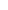(P:(SetProp).p:Setx:Set. (xpP(x))